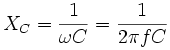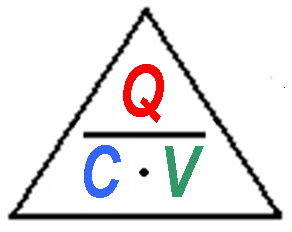Deutsche VersionCapacitive reactance  calculator XC

• Reactance of a capacitor

Calculate the reactance XC. Choosing a capacitor C and frequency f is best.
Capacitive reactance (symbol XC) is a measure of a capacitor's opposition to
AC (alternating current). Like resistance it is measured in ohms, but
reactance is more complex than resistance because its value depends on the
frequency (
f) of the electrical signal passing through the capacitor as well
as on the capacitance,
C.
Capacitive reactance, XC = 1 / (2 × π × f × C)
where:
XC = reactance in ohms (ohm), f = frequency in hertz (Hz), C = capacitance in farads (F)

The reactance XC is large at low frequencies and small at high frequencies.
For steady DC which is zero frequency,
XC is infinite (total opposition),
hence the rule that capacitors pass AC but block DC.
For example a 1 µF capacitor has a reactance of 3.2 kΩ for a 50 Hz signal, but
when the frequency is higher at 10 kHz its reactance is only 16 Ω.

Capacitance C, frequency f, and capacitive reactance XC

Enter the values for capacitance and frequency, then press "calculate".

 Capacitance C and units microfarads nanofarads picofarads Frequency fc and units MHz kHz Hz ↓ Capacitive reactance XC ohms kiloohms megohms gigaohms

 Capacitive reactance is an opposition to the change of voltage across an element. Capacitive reactance XC is inversely proportional to the signal frequency f (or angular frequency ω) and the capacitance C.

Capacitive reactanceImpedanceReactance calculator of C and L

Frequency response and equalization (EQ filter) - Preemphasis and deemphasis

 1,000,000 pF = 1000 nF = 1 µF = 1 × 10−6 F   100,000 pF = 100 nF = 0.1 µF = 1 × 10−7 F     10,000 pF = 10 nF = 0.01 µF = 1 × 10−8 F       1,000 pF = 1 nF = 0.001 µF = 1 × 10−9 F         100 pF = 0.1 nF = 0.0001 µF = 1 × 10−10 F           10 pF = 0.01 nF = 0.00001 µF = 1 × 10−11 F             1 pF = 0.001 nF = 0.000001 µF = 1 × 10−12 F

 SI multiples for hertz (Hz) Value Symbol Name 103 Hz kHz kilohertz 106 Hz MHz megahertz 109 Hz GHz gigahertz 1012 Hz THz terahertz Common prefixed units

Capacity of a capacitor (condenser) C = Q / V

Capacity C, charge Q, and voltage V.

Please enter two values, the third value will be calculated.

 Capacity C faradsCharge Q coulombs Voltage V volts

RC Filter and Cutoff Frequency

 A typical question: "How to measure a capacitor?" http://lmgtfy.com/?q=How+to+measure+a+capacitor&filter=0